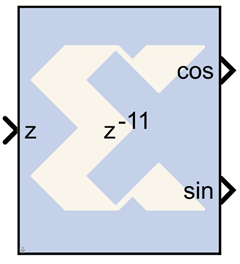# CORDIC SINCOS - 2020.2 English

## Vivado Design Suite Reference Guide: Model-Based DSP Design Using System Generator (UG958)

Document ID
UG958
Release Date
2020-11-18
Version
2020.2 English

The Xilinx CORDIC SINCOS reference block implements Sine and Cosine generator circuit using a fully parallel CORDIC (COordinate Rotation DIgital Computer) algorithm in Circular Rotation mode.That is, given input angle z, it computes the output cosine (z) and sine (z). The CORDIC processor is implemented using building blocks from the Xilinx blockset. The CORDIC sine cosine algorithm is implemented in the following 3 steps:

1. Coarse Angle Rotation - The algorithm converges only for angles between -pi/2 and pi/2. If z > pi/2, the input angle is reflected to the 1st quadrant by subtracting pi/2 from the input angle. When z < -pi/2, the input angle is reflected back to the 3rd quadrant by adding pi/2 to the input angle. The sine cosine circuit has been designed to converge for all values of z, except for the most negative value.
2. Fine Angle Rotation - By setting x equal to 1/1.646760 and y equal to 0, the rotational mode CORDIC processor yields cosine and sine of the input angle z.
3. Co-ordinate Correction - If there was a reflection applied in Step 1, this step applies the appropriate correction.

For z > pi/2: using z = t + pi/2, then

sin (z ) = sin(t).cos(pi/2) + cos(t).sin(pi/2) = cos(t)
cos (z ) = cos(t).cos(pi/2) - sin(t).sin(pi/2) = -sin(t)

For z < pi/2: using z = t - pi/2, then

sin (z ) = sin(t).cos(-pi/2) + cos(t).sin(-pi/2) = -cos(t)
cos (z ) = cos(t).cos(-pi/2) - sin(t).sin(-pi/2) = sin(t)

## Block Parameters

The block parameters dialog box can be invoked by double-clicking the icon in your Simulink model.

Parameters specific to this reference block are as follows:

• Number of Processing Elements: specifies the number of iterative stages used for linear rotation.
• Input Data Width: specifies the width of the input z. The input z should be signed data type with the same data width as specified.
• Input Binary Point Position: specifies the binary point position for input z. The input z should be signed data type with the same binary point position. The binary point should be chosen to provide enough bits for representing pi/2.
• Latency for each Processing element: This parameter sets the pipeline latency after each iterative circular rotation stage. The latency of the CORDIC SINCOS block is calculated based on the formula specified as follows: Latency = 3 + sum (latency of Processing Elements)

## Reference

1) J. E. Volder, The CORDIC Trigonometric Computing Technique, IRE Trans. On Electronic Computers, Vol. EC-8, 1959, pp. 330-334. 2) J. S. Walther, A Unified Algorithm for Elementary Functions, Spring Joint Computer Conference (1971) pp. 379-385. 3) Yu Hen Hu, CORDIC-Based VLSI Architectures for Digital Signal Processing, IEEE Signal Processing Magazine, pp. 17-34, July 1992.